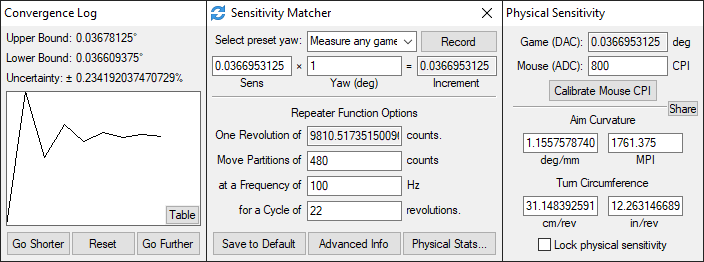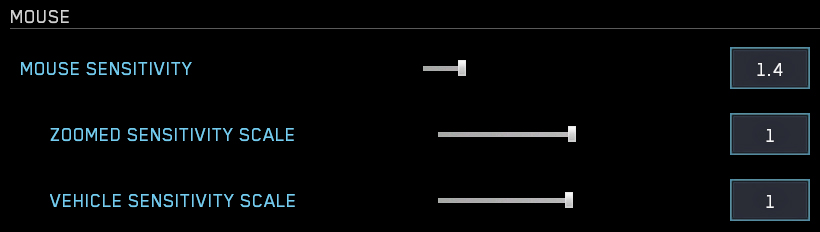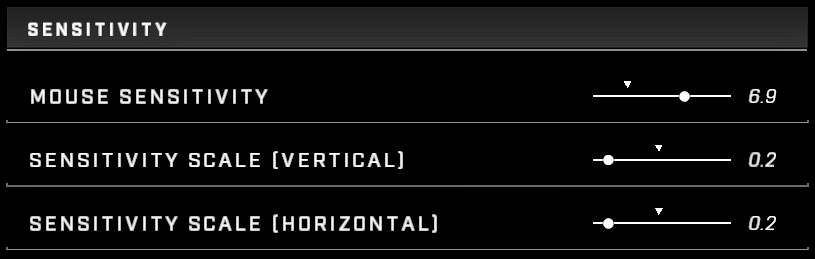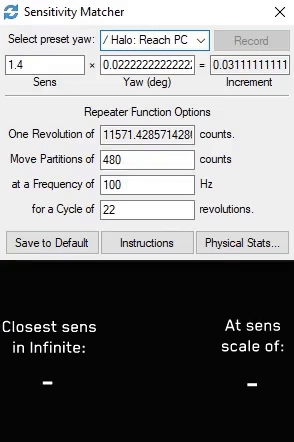# Convert Halo MCC mouse sensitivity to Halo InfiniteWe'll use KovaaK's Sensitivity Matcher to convert our sensitivity.

## Yaw Presets For Halo Infinite

KovaaKs Sensitivity Matcher helps you find the mouse sensitivity yaw value for every game.

Halo MCC uses a yaw value of 0.02222222222222222222222222222222

Halo Infinite uses a yaw value of 0.0225 (1.0 sensitivity scaling)

I've created preset yaw value profiles for Halo Infinite's 20 different options for mouse sensitivity scaling.

The presets are:

[Halo Infinite 0.1] yaw=0.00225 [Halo Infinite 0.2] yaw=0.0045 [Halo Infinite 0.3] yaw=0.00675 [Halo Infinite 0.4] yaw=0.009 [Halo Infinite 0.5] yaw=0.01125 [Halo Infinite 0.6] yaw=0.0135 [Halo Infinite 0.7] yaw=0.01575 [Halo Infinite 0.8] yaw=0.018 [Halo Infinite 0.9] yaw=0.02025 [Halo Infinite 1.0] yaw=0.0225 [Halo Infinite 1.1] yaw=0.02475 [Halo Infinite 1.2] yaw=0.027 [Halo Infinite 1.3] yaw=0.02925 [Halo Infinite 1.4] yaw=0.0315 [Halo Infinite 1.5] yaw=0.03375 [Halo Infinite 1.6] yaw=0.036 [Halo Infinite 1.7] yaw=0.03825 [Halo Infinite 1.8] yaw=0.0405 [Halo Infinite 1.9] yaw=0.04275 [Halo Infinite 2.0] yaw=0.045

To import these values into KovaaK's Sensitivity Matcher, follow these steps:

Navigate to the install location of KovaaK's Sensitivity Matcher

Paste all of the yaw value profiles shown above into your UserSettings.ini file

Save the file and open SensitivityMatcher.exe. You should now have all of the custom Halo Infinite yaw presets in Sensitivity Matcher.

## Step-by-step Sens Conversion TutorialTo convert your sensitivity from MCC to Halo Infinite, follow these steps:

In MCC, find your mouse sensitivity value (Options & Career > Settings > Controls > Configure mouse & keyboard (select) > Mouse sensitivity)

In Halo Infinite, find your mouse sensitivity scaling value (Control Panel (F1) > Settings > Keyboard/Mouse > Sensitivity scale. I suggest having both vertical and horizontal scale be the same

In Sensitivity Matcher, select the preset yaw "Halo: Reach PC" and input your MCC sensitivity in the "Sens" -field (The 0.022222... yaw value is used in all Halo titles in MCC, not just Reach)

Select the "Halo Infinite x.x" preset yaw profile according to your sensitivity scaling in Halo Infinite and your converted sensitivity will be displayed in the "Sens" -field.

If you insist on not downloading KovaaK's Sensitivity Matcher, you can do a few simple calculations to get your MCC sens converted to Infinite's.

● Multiply your MCC sens by 0.02222222222222222222222222222222

1.4 * 0.02222222222222222222222222222222 = 0.0311111111111111 ● Divide the output value by the yaw value for the Halo Infinite sensitivity scale of your choosing (0.2 sensitivity scale for this example)

0.0311111111111111 / 0.0045 = 6.91358024691358 ● The final output value is the sensitivity to use in Halo Infinite along with the sensitivity scale that you used for the calculation.

## Important NoticesImportant things to note:

Due to sensitivity value input field being so bad in Halo Infinite, you should use the smallest sensitivity scaling to get the most gradual adjustment of your sensitivity. Watch this video by XLR8 explaining sensitivity scaling to understand more.

In Sensitivity Matcher, starting from the "Halo Infinite 0.1" yaw preset and going up in 0.1 increments, pick a sensitivity scale yaw preset that makes your converted sensitivity as close to a 1 decimal accuracy as possible.

What I mean by this is don't choose a converted sens value of e.g. 3.45679012345679 (with 0.4 sensitivity scale), since the most accurate value you can input in Halo Infinite is either 3.4 or 3.5. Rather pick a value like 2.30452674897119 (with 0.6 sensitivity scale) so you can make your sensitivity in Halo Infinite be 2.3 with 0.6 sensitivity scale to have it be a closer match to the MCC sensitivity (1.4 in this example).

## TLDR, In Practice Example

In practice:

I choose the "Halo: Reach PC" preset yaw profile in Sensitivity Matcher and input my MCC sensitivity of 1.4 in the "Sens" -field

I then go through the preset yaw profiles starting from "Halo Infinite 0.1"

Since with a 0.1 scale, the converted sensitivity (13.8271604938272) is above 10, which is the maximum that Halo Infinite allows the value to be, I choose the next yaw profile being "Halo Infinite 0.2", which gives me a sens of 6.91358024691358. Since this value is a very close value to 6.9 - a sensitivity value of one decimal accuracy, that I can input in Halo Infinite - and uses a low sensitivity scale, meaning that making adjustments to the sensitivity is more gradual, I will choose this sensitivity and put my sensitivity in Halo Infinite to 6.9 and my Sensitivity Scale to 0.2 in both vertical and horizontal fields.

## Feedback

Send me your feedback and issues that you found in the comments!

Thanks for viewing!

`Source: https://steamcommunity.com/sharedfiles/filedetails/?id=2794288620					`